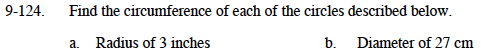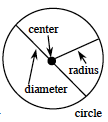### Home > MC1 > Chapter 9 > Lesson 9.3.1 > Problem9-124

9-124.Recall the diagram from the Math Notes boxfrom Lesson 9.3.1 below.
Also recall that the same Math Notes box says that the circumference = 2π(radius).2π(3inches) = 6π ≈ 18.84 inches

Refer to the diagram from part (a) and recall that the circumference = π(diameter).

27π
To get an approximate decimal answer, round π so that it equals 3.14.